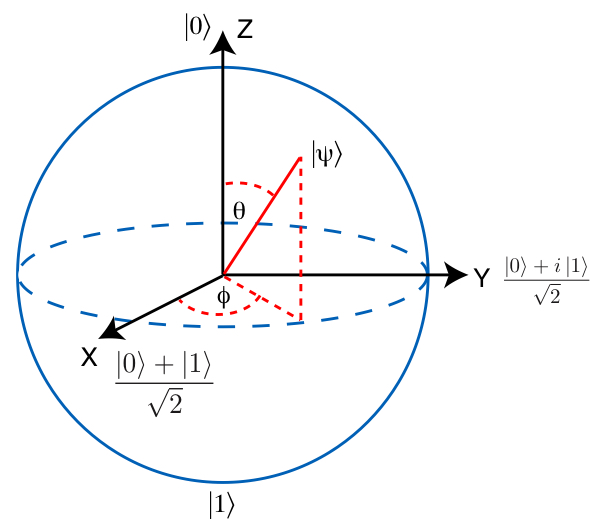# Qubit basis states

## Single-qubit computational basis states

The two orthogonal z-basis states of a qubit are defined as:

• $\vert 0\rangle$
• $\vert 1\rangle$

When we talk about the qubit basis states we implicitly refer to the z-basis states as the computational basis states.

The two orthogonal x-basis states are:
$\vert +\rangle =\frac{\vert 0\rangle + \vert 1\rangle}{\sqrt{2}}$ $\vert -\rangle =\frac{\vert 0\rangle - \vert 1\rangle}{\sqrt{2}}$

The two orthogonal y-basis states are:
$\vert R\rangle =\frac{\vert 0\rangle + \imath \vert 1\rangle}{\sqrt{2}}$ $\vert L\rangle =\frac{\vert 0\rangle - \imath \vert 1\rangle}{\sqrt{2}}$

The basis states are located at opposite points on the Bloch sphere:Bloch sphere courtesy of http://www.laborsciencenetwork.com

## Multi-qubit computational basis states

A single-qubit has two computational basis states. In the z-basis these are $\vert 0 \rangle$ and $\vert 1 \rangle$. A two-qubit system has 4 computational basis states denoted as $\vert 00 \rangle$, $\vert 01 \rangle$, $\vert 10 \rangle$, $\vert 11 \rangle$.

A multi-qubit system of N qubits has $2 ^{N}$ computational basis states denoted as $\vert 00...00 \rangle$, $\vert 00 \cdots 01 \rangle$, $\vert 00 \cdots 10 \rangle$ ... $\vert 11 \cdots 11 \rangle$.

## Probability amplitudes

Associated with each computational basis state is a probability amplitude $\alpha_{i}$, which is a complex number.

As an example, a system of three qubits is described by the expression:

$\lvert \Psi \rangle = \alpha_{0} \lvert 000 \rangle + \alpha_{1} \lvert 001 \rangle + \alpha_{2} \lvert 010 \rangle + \cdots + \alpha_{7} \lvert 111 \rangle$

where $\alpha_{i}$ are the probability amplitudes associated to the computational basis states.

## Initialization and measurement bases

By default, all qubits are initialized in the $|0\rangle$ state in the z-basis.

State initialization in a specific basis can be done explicitly with the cQASM instructions prep_z, prep_y and prep_x, which prepare qubits in the $\vert 0 \rangle$, $\vert R \rangle$ and $\vert + \rangle$ states respectively.

By default, qubits are measured with the measure or measure_all instruction in the z-basis.
Qubit measurement in a specific basis can be done explicitly with the cQASM instructions measure_x, measure_y and measure_z.

## Declared states

• When a qubit is in the $\vert 0 \rangle$ state ($\vert 1 \rangle$ state), a measurement in the z-basis will result in 0 (1)
• When a qubit is in the $\vert R \rangle$ state ($\vert L \rangle$ state), a measurement in the y-basis will result in 0 (1)
• When a qubit is in the $\vert + \rangle$ state ($\vert - \rangle$ state), a measurement in the x-basis will result in 0 (1)

$\vert R \rangle$ and $\vert L \rangle$ stand for Right and Left. Other notations that are often used for these states are $\vert \imath \rangle$ and $\vert - \imath \rangle$.